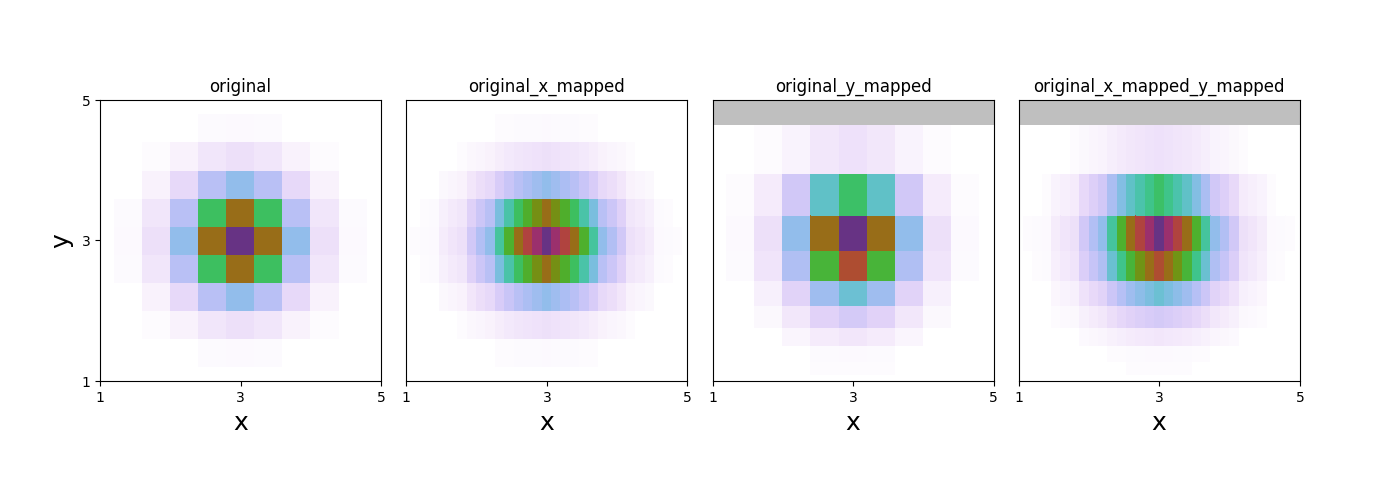# Map-Variable

An example of how map-variable works.Out:

```data mapped from (11, 11) to (31, 11)
data mapped from (11, 11) to (11, 11)
data mapped from (31, 11) to (31, 11)
```

```import numpy as np
from matplotlib import pyplot as plt
import WrightTools as wt

# create original arrays
x = np.linspace(1, 5, 11)[:, None]
y = np.linspace(1, 5, 11)[None, :]
arr = np.exp(-1 * (((x - 3) / 0.6) ** 2 + ((y - 3) / 0.6) ** 2))
# create data object
d = wt.data.Data(name="original")
d.create_variable("x", values=x)
d.create_variable("y", values=y)
d.create_channel("z", arr)
d.transform("x", "y")
# create new data objects
pointsx = np.linspace(x.min(), x.max(), 31)  # linear spacing
pointsy = points = np.logspace(0, 0.7, 11)  # log spacing
dx = d.map_variable("x", points=pointsx)  # just linear along x
dy = d.map_variable("y", points=pointsy)  # just log along y
dxy = dx.map_variable("y", points=pointsy)  # linear along x and log along y
ds = [d, dx, dy, dxy]
# create figure
fig, gs = wt.artists.create_figure(width="double", cols=[1, 1, 1, 1])
for i, d in enumerate(ds):
ax = plt.subplot(gs[i])
ax.pcolor(d)
ax.set_title(d.natural_name)
ax.set_xlim(1, 5)
ax.set_ylim(1, 5)
ticks = [1, 3, 5]
wt.artists.set_fig_labels(
xlabel=d.axes.label, ylabel=d.axes.label, xticks=ticks, yticks=ticks
)
```

Total running time of the script: ( 0 minutes 0.481 seconds)

Gallery generated by Sphinx-Gallery